JEE  >  VITEEE Maths Test - 10

# VITEEE Maths Test - 10

Test Description

## 40 Questions MCQ Test VITEEE: Subject Wise and Full Length MOCK Tests | VITEEE Maths Test - 10

VITEEE Maths Test - 10 for JEE 2023 is part of VITEEE: Subject Wise and Full Length MOCK Tests preparation. The VITEEE Maths Test - 10 questions and answers have been prepared according to the JEE exam syllabus.The VITEEE Maths Test - 10 MCQs are made for JEE 2023 Exam. Find important definitions, questions, notes, meanings, examples, exercises, MCQs and online tests for VITEEE Maths Test - 10 below.
Solutions of VITEEE Maths Test - 10 questions in English are available as part of our VITEEE: Subject Wise and Full Length MOCK Tests for JEE & VITEEE Maths Test - 10 solutions in Hindi for VITEEE: Subject Wise and Full Length MOCK Tests course. Download more important topics, notes, lectures and mock test series for JEE Exam by signing up for free. Attempt VITEEE Maths Test - 10 | 40 questions in 50 minutes | Mock test for JEE preparation | Free important questions MCQ to study VITEEE: Subject Wise and Full Length MOCK Tests for JEE Exam | Download free PDF with solutions
 1 Crore+ students have signed up on EduRev. Have you?
VITEEE Maths Test - 10 - Question 1

### If x +y = 60; x, y > 0, then maximum value of xy3 is

VITEEE Maths Test - 10 - Question 2

### The area bounded by the x-axis and the curve y = 4x - x2 - 3 is

VITEEE Maths Test - 10 - Question 3

### If R → R is continuous such that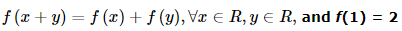then f(100) =

VITEEE Maths Test - 10 - Question 4

The value of arg [(1-i√3)/(1+i√3)] is

VITEEE Maths Test - 10 - Question 5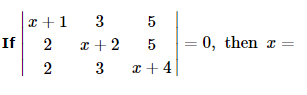VITEEE Maths Test - 10 - Question 6

(x + y + z) (x + y ω + z ω2) (x + yω2 + z ω)   =

VITEEE Maths Test - 10 - Question 7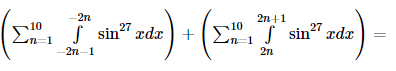VITEEE Maths Test - 10 - Question 8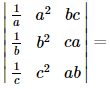Detailed Solution for VITEEE Maths Test - 10 - Question 8

Multiply R1, R2, R3 by a, b, c respectively and divide by abc
Take abc common from C3 so that C1, C3 are identical

VITEEE Maths Test - 10 - Question 9
The differential equation with respect to the curve y=emx is
VITEEE Maths Test - 10 - Question 10

Which equation has the solution y=A sinx+B cosx?

VITEEE Maths Test - 10 - Question 11

The solution of the differential equation x2 dy/dx-xy=1+cos y/x is

VITEEE Maths Test - 10 - Question 12

Let f(x) = log |x - 1|, x ≠ 1. The value of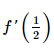is

Detailed Solution for VITEEE Maths Test - 10 - Question 12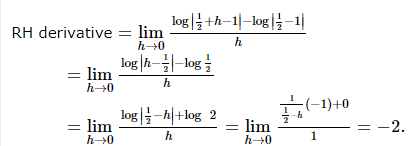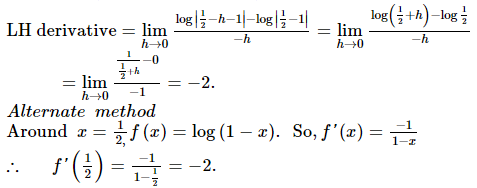VITEEE Maths Test - 10 - Question 13

The solution of the equation (1+x2)(1+y)dy+(1+x)(1+y2)dx=0 is

VITEEE Maths Test - 10 - Question 14

(d/dx)[cos(1-x2)2]=

VITEEE Maths Test - 10 - Question 15

If f x = log x , g x = x3 then f[ga]+f[gb]

=

VITEEE Maths Test - 10 - Question 16

If ∫(sin 2x - cos 2x)dx = (1/√2) sin (2x-a) + b

VITEEE Maths Test - 10 - Question 17

If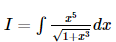then I is equal to

VITEEE Maths Test - 10 - Question 18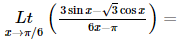VITEEE Maths Test - 10 - Question 19

The valuie of sin-1 cos(sin-1 x) + cos-1 sin (cos-1 x) is

VITEEE Maths Test - 10 - Question 20

True statement for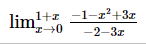VITEEE Maths Test - 10 - Question 21

Consider the sentence: x < 5
Which of the following integers makes this open sentence true?

Detailed Solution for VITEEE Maths Test - 10 - Question 21

The only number less than 5 in the list of answers is 4.

VITEEE Maths Test - 10 - Question 22

If |A| represents the determinant of a square matrix of order 3 then (-2A)=

VITEEE Maths Test - 10 - Question 23

If B is a non-singular matrix and A is a square matrix, then det (B-1AB) =

VITEEE Maths Test - 10 - Question 24

Five seats are vacant in a railway compartment, then in how many ways can three passengers be seated on these seats?

VITEEE Maths Test - 10 - Question 25

Two dice are thrown simultaneously. The probability of obtaining a total score of seven is

Detailed Solution for VITEEE Maths Test - 10 - Question 25

There are six possible ways as to the number of points on the first die; and to each of these ways, there corresponding 6 possible numbers of points on second die.
Hence total number of ways S = 6 x 6 = 36
We now find out how many ways are favorable to the total of 7 points.
This may happen only in following ways:
(1, 6), (6, 1), (2, 5), (5, 2), (3, 4), (4, 3).
Hence, required Probability = 6/36 =1/6.

VITEEE Maths Test - 10 - Question 26

If S is a sample space,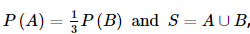, where A, B are two mutually exclusive events, then P(A) =

VITEEE Maths Test - 10 - Question 27

In a Poisson distribution mean is 16, then S.D is

VITEEE Maths Test - 10 - Question 28

In Δ A B C , i f A = 600then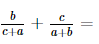VITEEE Maths Test - 10 - Question 29

113 + 123 + 133 + ... + 203 is

VITEEE Maths Test - 10 - Question 30

For a frequency distribution mean deviation from mean is computed by

VITEEE Maths Test - 10 - Question 31

The average weight of students in a class of 35 students is 40 kg. If the weight of the teacher be included, the average rises by (1/2) kg ; the weight of the teacher is

VITEEE Maths Test - 10 - Question 32

If x = 2 + i √3, then the value of, 4x2 + 8x + 13 is____

Detailed Solution for VITEEE Maths Test - 10 - Question 32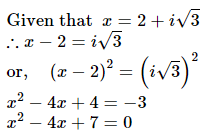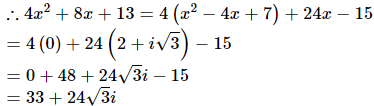VITEEE Maths Test - 10 - Question 33

A quadratic equation whose one root is the square root of -47+8√-3 is

VITEEE Maths Test - 10 - Question 34

The intersection of the spheres x2 + y2 + z2 + 7x - 2y - z = 13 and x2 + y2 + z2 - 3x + 3y + 4z = 8 is the same as the intersection of one of the sphere and the plane

VITEEE Maths Test - 10 - Question 35

The smallest values of θ satisfying the equation √ 3 cot θ + tan θ = 4 is

VITEEE Maths Test - 10 - Question 36

A tetrahedron has vertices at O (0, 0, 0), A(1, 2, 1), B(2, 1, 3) and C (-1, 1, 2). Then the angle between the faces OAB and ABC will be

VITEEE Maths Test - 10 - Question 37
The orthocentre of the triangle whose vertices are (5, -2), (-1, 2) and (1, 4) is
VITEEE Maths Test - 10 - Question 38

The angle between the two straight lines represented by 6y2 - xy - x2 + 30y + 36 = 0 is

VITEEE Maths Test - 10 - Question 39

Let a, b and c be distributed non-vegetative numbers. If the vectors aî + aĵ + ck̂, î + k̂ and cî̂ + cĵ̂ + bk̂ lie in a plane, then c is

VITEEE Maths Test - 10 - Question 40

If vectors i+2j+3k and 3i-2j+k represents the adjacent sides of a parallelogram, the area of parallelogram is

## VITEEE: Subject Wise and Full Length MOCK Tests

1 videos|3 docs|73 tests
 Use Code STAYHOME200 and get INR 200 additional OFF Use Coupon Code
Information about VITEEE Maths Test - 10 Page
In this test you can find the Exam questions for VITEEE Maths Test - 10 solved & explained in the simplest way possible. Besides giving Questions and answers for VITEEE Maths Test - 10, EduRev gives you an ample number of Online tests for practice

## VITEEE: Subject Wise and Full Length MOCK Tests

1 videos|3 docs|73 tests